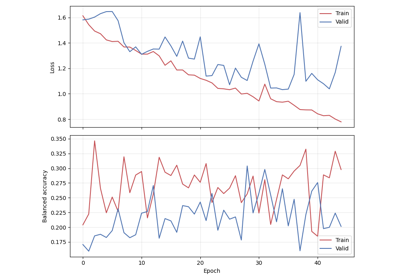# braindecode.models.USleep#

class braindecode.models.USleep(in_chans=2, sfreq=128, depth=12, n_time_filters=5, complexity_factor=1.67, with_skip_connection=True, n_classes=5, input_size_s=30, time_conv_size_s=0.0703125, ensure_odd_conv_size=False, apply_softmax=False)[source]#

Sleep staging architecture from Perslev et al 2021.

U-Net (autoencoder with skip connections) feature-extractor for sleep staging described in .

For the encoder (‘down’):

– the temporal dimension shrinks (via maxpooling in the time-domain) – the spatial dimension expands (via more conv1d filters in the

time-domain)

For the decoder (‘up’):

– the temporal dimension expands (via upsampling in the time-domain) – the spatial dimension shrinks (via fewer conv1d filters in the

time-domain)

Both do so at exponential rates.

Parameters
• in_chans (int) – Number of EEG or EOG channels. Set to 2 in  (1 EEG, 1 EOG).

• sfreq (float) – EEG sampling frequency. Set to 128 in .

• depth (int) – Number of conv blocks in encoding layer (number of 2x2 max pools) Note: each block halve the spatial dimensions of the features.

• n_time_filters (int) – Initial number of convolutional filters. Set to 5 in .

• complexity_factor (float) – Multiplicative factor for number of channels at each layer of the U-Net. Set to 2 in .

• with_skip_connection (bool) – If True, use skip connections in decoder blocks.

• n_classes (int) – Number of classes. Set to 5.

• input_size_s (float) – Size of the input, in seconds. Set to 30 in .

• time_conv_size_s (float) – Size of the temporal convolution kernel, in seconds. Set to 9 / 128 in .

• ensure_odd_conv_size (bool) – If True and the size of the convolutional kernel is an even number, one will be added to it to ensure it is odd, so that the decoder blocks can work. This can ne useful when using different sampling rates from 128 or 100 Hz.

• apply_softmax (bool) – If True, apply softmax on output (e.g. when using nn.NLLLoss). Use False if using nn.CrossEntropyLoss.

References

1(1,2,3,4,5,6,7)

Perslev M, Darkner S, Kempfner L, Nikolic M, Jennum PJ, Igel C. U-Sleep: resilient high-frequency sleep staging. npj Digit. Med. 4, 72 (2021). perslev/U-Time

Methods

forward(x)[source]#

If input x has shape (B, S, C, T), return y_pred of shape (B, n_classes, S). If input x has shape (B, C, T), return y_pred of shape (B, n_classes).

## Examples using `braindecode.models.USleep`#Sleep staging on the Sleep Physionet dataset using U-Sleep network

Sleep staging on the Sleep Physionet dataset using U-Sleep network